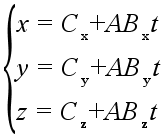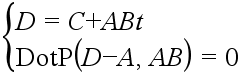# How to find the intersection point of two lines?

Okay, so the blue line right here is determined by the position of point C, and is parallel to the line AB (in red)
Now I’m trying to find the yellow ray, as well as that teal point which is the intersection point between the yellow and blue rays.
Does anyone have any clue?
In other words, I’m trying to find on which point on the blue line will a line from point A to that point be perpendicular to line AB (the red line).

I would expect that you can do this with RayCasting. Just fire the ray vertically:

``````local RaycastResult = workspace:Raycast(rayPosition, rayDirection, rayParams)
``````

This returns an variety of data you can use to determine the intersection point. See:

Wouldn’t the yellow ray just be a ray in upwards direction from point A?

Edit: And the intersection point would be point A’s position but height changed to the height of point C.

So basically, you need to find the point closest to `A` on the line that goes through `C`. If `A` and `B` have the same y-coordinate and `C` is directly above the line `AB`, @Soflowsen’s suggestion will work. Otherwise you may need a slightly more complicated approach that is explained below.

I’ll call the teal point `D`. Because the line that goes through `C` is parallel to line `AB`, they both have the vector `AB = B - A` as their direction vector. Here’s the parametric equation of the line that goes through `C`:Any point `Q` on the line that goes through `C` can be written as `Q = C + ABt`, where `t` is a real number. We need to calculate such a value for `t` that we get a point `D = C + ABt` that satisfies the condition `(D-A):Dot(AB) = 0`, because the dot product of perpendicular vectors is `0`.

So we can form two vector equations:Using the parametric equation, the two equations above can be combined into one equation.

By solving this equation we get a formula for `t`.

Here’s a function for calculating the point.

``````local function GetClosestPointToAOnALineThroughCParallelToLineAB(A, B, C)
local AB = B - A
local t = (AB.X * (A.X - C.X) + AB.Y * (A.Y - C.Y) + AB.Z * (A.Z - C.Z)) / (AB.X^2 + AB.Y^2 + AB.Z^2)
local D = C + AB * t
return D
end``````
1 Like

There was a thread like this a long time ago, here’s the link to it:

PlaasBoer’s solution is the best one in my opinion.